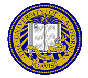# Outline for May 7, 1997

1. Greetings and Felicitations
2. HRU result and proof
1. General result (HRU Theorem): Whether a given state of a given protection system is safe for a given generic right is undecidable
2. Proof approach: reduce halting problem to safety problem
3. Proof: T is an arbitrary Turing machine; encode T's state as an ACM and the head moves as commands of the protection system
T in state q has scanned k cells; cells k+1, ... are blank. Represent q as an ACM with k subjects and objects meeting the following:
1. If cell s of tape contains symbol X, then A[s, s] contains the right X
2. A[s, s+1] contains the right Own; this induces an ordering on the subjects according to the symbols on the tape; note Own is above main diagonal
3. A[k, k] contains the right End (signalling the farthest right the head has moved)
4. If tape head at cell s, A[s, s] contains the right q
Initially, q0 is an ACM with one subject and one object corresponding to the first tape cell.
Move: d(q, X) = (p, Y, L) means in state q and head scanning symbol X, changes state to p, overwrites X with Y, and moves head left (R would be right). Moves represented as commands; example: above move is:
command cqX(s, s')
if Own in A[s, s'] and
q in A[s', s'] and
X in A[s', s']
then
delete q from A[s', s']
delete X from A[s', s']
enter Y into A[s', s']
enter p into A[s', s']
end.
where s' is current position of tape head and s is square to left. A move to the right has to handle the End case; 2 different commands, appropriately conditioned
Now, if T reaches its final state qf, then right qf leaked into some position of ACM; equivalently, if T halts, the right qf is leaked. So if the safety question is decideable, so is the halting problem, which is false; so the safety problem is undecidable.
3. Take-Grant: rules, initial and terminal spans, bidirectionality of t and g given subject-only graph

Notes by Michael Clifford: [Text]
You can get this document in Postscript, ASCII text, or Framemaker version 5.1.Send email to cs253@csif.cs.ucdavis.edu.

Department of Computer Science
University of California at Davis
Davis, CA 95616-8562